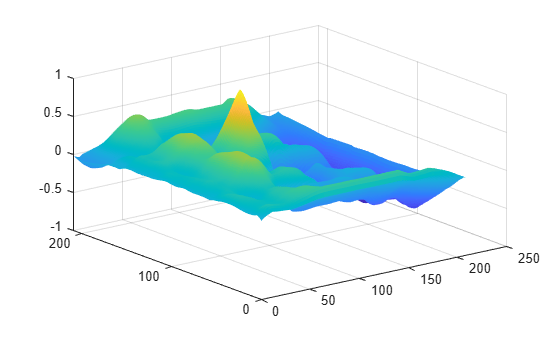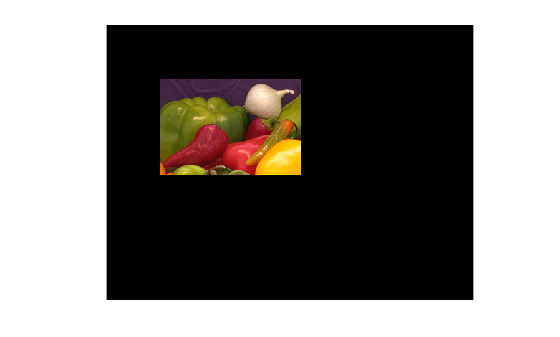Documentation

Registering an Image Using Normalized Cross-Correlation

This example shows how to find a template image within a larger image. Sometimes one image is a subset of another. Normalized cross-correlation can be used to determine how to register or align the images by translating one of them.

Step 1: Read Image

imshow(onion)figure, imshow(peppers)Step 2: Choose Subregions of Each Image

It is important to choose regions that are similar. The image sub_onion will be the template, and must be smaller than the image sub_peppers. You can get these sub regions using either the non-interactive script below or the interactive script.

% non-interactively
rect_onion = [111 33 65 58];
rect_peppers = [163 47 143 151];
sub_onion = imcrop(onion,rect_onion);
sub_peppers = imcrop(peppers,rect_peppers);

% OR

% interactively
%[sub_onion,rect_onion] = imcrop(onion); % choose the pepper below the onion
%[sub_peppers,rect_peppers] = imcrop(peppers); % choose the whole onion

% display sub images
figure, imshow(sub_onion)figure, imshow(sub_peppers)Step 3: Do Normalized Cross-Correlation and Find Coordinates of Peak

Calculate the normalized cross-correlation and display it as a surface plot. The peak of the cross-correlation matrix occurs where the sub_images are best correlated. normxcorr2 only works on grayscale images, so we pass it the red plane of each sub image.

c = normxcorr2(sub_onion(:,:,1),sub_peppers(:,:,1));
figure, surf(c), shading flatStep 4: Find the Total Offset Between the Images

The total offset or translation between images depends on the location of the peak in the cross-correlation matrix, and on the size and position of the sub images.

% offset found by correlation
[max_c, imax] = max(abs(c(:)));
[ypeak, xpeak] = ind2sub(size(c),imax(1));
corr_offset = [(xpeak-size(sub_onion,2))
(ypeak-size(sub_onion,1))];

% relative offset of position of subimages
rect_offset = [(rect_peppers(1)-rect_onion(1))
(rect_peppers(2)-rect_onion(2))];

% total offset
offset = corr_offset + rect_offset;
xoffset = offset(1);
yoffset = offset(2);

Step 5: See if the Onion Image was Extracted from the Peppers Image

Figure out where onion falls inside of peppers.

xbegin = round(xoffset+1);
xend   = round(xoffset+ size(onion,2));
ybegin = round(yoffset+1);
yend   = round(yoffset+size(onion,1));

% extract region from peppers and compare to onion
extracted_onion = peppers(ybegin:yend,xbegin:xend,:);
if isequal(onion,extracted_onion)
disp('onion.png was extracted from peppers.png')
end
onion.png was extracted from peppers.png

Step 6: Pad the Onion Image to the Size of the Peppers Image

Pad the onion image to overlay on peppers, using the offset determined above.

recovered_onion = uint8(zeros(size(peppers)));
recovered_onion(ybegin:yend,xbegin:xend,:) = onion;
figure, imshow(recovered_onion)Step 7: Use Alpha Blending To Show Images Together

Display one plane of the peppers image with the recovered_onion image using alpha blending.

figure, imshowpair(peppers(:,:,1),recovered_onion,'blend')